# Topological group

A seton which two structures are given — a group structure and a topology, such that the group operations are continuous. Specifically, the mapping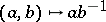from the direct product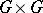intomust be continuous. A subgroupof a topological groupis a topological group in the induced topology. The quotient space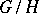of cosets is given the quotient topology with respect to the canonical mapping fromonto. Ifis a normal subgroup of, then(the quotient group ofby) is a topological group.

Examples of topological groups: the vector groupis the direct product ofcopies of the additive groupof real numbers with the natural topology; the circle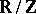is the quotient group ofby the subgroup of integers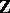; every Lie group; any abstract group with the discrete topology; an arbitrary topological vector space.

Usually the underlying space of a topological group is assumed to be Hausdorff. The quotient spaceis Hausdorff if and only if the subgroupis closed in(from now on all subgroups considered are assumed to be closed). The quotient spaceof cosets is regular. However, there exist topological groups with non-normal underlying space (cf. ).

A topological group is said to be connected, totally disconnected, compact, locally compact, etc., if the corresponding property holds for its underlying topological space. The connected component of the identity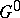is the largest connected closed subgroup of. The quotient group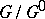is totally disconnected. A locally compact totally-disconnected group has an open compact subgroup. Ifis a compact totally-disconnected group, then every neighbourhood of the identity contains an open normal subgroup of. Hence it follows that the class of compact totally-disconnected groups coincides with the class of profinite groups (cf. Profinite group). These play an important role in Galois theory, where, with the Krull topology, they appear as the Galois groups of infinite separable fields.

Every topological group is a uniform space in a natural way. Specifically, a left uniform group structure on a topological groupis defined by the collection of setswhereruns over a system of neighbourhoods of the identity in; a right uniform structure is defined by symmetry. The topology arising from the uniform structure is the same as the original topology on the group. The existence of a uniform structure on a topological group allows one to introduce and apply the notions of uniform continuity (for example, for real-valued functions on a topological group), Cauchy sequences, completeness, and completion. A locally compact topological group is complete in its uniform structure. A consequence of this is the fact that any locally compact subgroup of a Hausdorff topological group is closed. There exist, however, topological groups which cannot even be imbedded in complete groups.

On every locally compact topological groupthere exists a non-trivial measurethat is invariant under left translation (that is, for every-measurable subset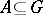and every elementthe subset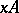is measurable and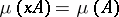). Such a measure is called a Haar measure. It is unique up to multiplication by a constant.

If the topological groupis compact, then a Haar measure is also invariant under right translation. Moreover, in this case one may choose the constant factor such that. This enables one to consider the integralas the mean value of the functionon. The most important applications of Haar measure are concerned with the theory of continuous representations. Integration with respect to a Haar measure allows one to transfer to compact groups a significant part of the theory of representations of finite groups (for example, the orthogonality relation for characters, or for matrix entries), and also the Peter–Weyl theorem, which was first obtained for Lie groups. A consequence of this theorem is the fact that every compact group admits a complete system of finite-dimensional unitary representations (in other words, for every non-trivial element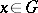there exists a representationsuch that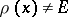). There exist locally compact groups without non-trivial finite-dimensional representations.

In essence, significant results about the structure of topological groups are known only for locally compact groups. For locally compact Abelian groups there is the following fundamental structure theorem: Every locally compact Abelian groupcan be represented as the direct product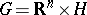, whereis a group with an open compact subgroup. This result is a consequence of a duality theory for locally compact Abelian groups (cf. Pontryagin duality). This theorem reduces the study of the structure ofto questions about the discrete groups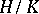and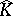, whereis the character group of, in other words, to questions in abstract group theory.

A definitive part in the development of the theory of topological groups was played by Hilbert's fifth problem. It was formulated in 1900 as a problem about local transformation groups, and was essential to the development of the theory of topological groups. The following has become the generally accepted formulation of it: Is every locally Euclidean topological group a Lie group? (A topological group is called locally Euclidean if it has a neighbourhood of the identity that is homeomorphic to a Euclidean space, i.e. if it is a topological manifold.) Hilbert's fifth problem was solved in 1952 (cf. ). An essential ingredient was the proof of the following criterion for being of Lie type: A locally compact groupis a Lie group if and only if it is a group without small subgroups (that is, if there exists a neighbourhood of the identity that does not contain a non-trivial subgroup). It was also shown that a locally compact groupwith compact quotient group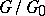is a projective limit of Lie groups (or, equivalently, every neighbourhood of the identity incontains a normal subgroupsuch thatis a Lie group). Every neighbourhood of the identity in an arbitrary locally compact group contains an open set of the form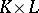, whereis a compact subgroup andis a connected local Lie group.

The fact that locally compact groups with compact quotient groupare projective limits of Lie groups made it possible to carry over many results previously known for Lie groups to this class of groups (cf. ). For example, every compact subgroup ofis contained in a maximal compact subgroup, and any two maximal compact subgroups ofare conjugate. Moreover, ifis one of the maximal compact subgroups of, then there exists a set of one-parameter subgroups,, such that the mapping sendingto the product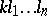is a homeomorphism from the group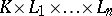onto.

After the solution of Hilbert's fifth problem, the major problem was to investigate in more detail the structure of locally compact groups satisfying some or other additional properties. Classes of groups satisfying some finiteness property have been studied; for example, the condition of finiteness of the special rank, different variants of the maximum and minimum conditions for subgroups, etc. (cf. ). A theory of locally nilpotent, locally compact groups arose. A large part of the results obtained here were later extended to the class of locally residually-nilpotent groups .